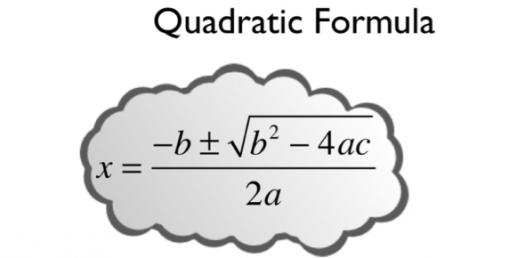Approved & Edited by ProProfs Editorial Team
The editorial team at ProProfs Quizzes consists of a select group of subject experts, trivia writers, and quiz masters who have authored over 10,000 quizzes taken by more than 100 million users. This team includes our in-house seasoned quiz moderators and subject matter experts. Our editorial experts, spread across the world, are rigorously trained using our comprehensive guidelines to ensure that you receive the highest quality quizzes.
| By Z3n8
Z
Z3n8
Community Contributor
Quizzes Created: 1 | Total Attempts: 164
Questions: 5 | Attempts: 168SettingsHere is a quiz about everything on my website.

• 1.

### Is this the Quadratic formula?

• A.

True

• B.

False

A. True
Explanation
The given statement "Is this the Quadratic formula?" is true.

Rate this question:

• 2.

### What Polynomial's graph looks like the graph below?

• A.

A line

• B.

A Parabola

• C.

An cubic curve

• D.

A graph of a cuartic polynomial

C. An cubic curve
Explanation
The graph below shows a curve that is not a straight line or a parabola, but it has a slight bend. This suggests that it could be a cubic curve. A cubic curve is a type of polynomial function with a degree of 3, meaning it has terms involving x raised to the power of 3, 2, 1, and a constant term. Therefore, it is reasonable to conclude that the graph below represents a cubic curve.

Rate this question:

• 3.

### What does the quadratic formula do?

• A.

It gives you the two roots to a quadratic equation

• B.

It gives you the roots to a linear equation

• C.

It tells you how many solutions an equation has.

• D.

It tells you what the meaning of life is.

A. It gives you the two roots to a quadratic equation
Explanation
The quadratic formula is a mathematical formula used to find the roots, or solutions, of a quadratic equation. A quadratic equation is an equation of the form ax^2 + bx + c = 0, where a, b, and c are constants. The quadratic formula states that the roots of a quadratic equation can be found using the formula x = (-b ± √(b^2 - 4ac))/(2a). This formula gives two values for x, which are the two roots of the quadratic equation. Therefore, the correct answer is that the quadratic formula gives you the two roots to a quadratic equation.

Rate this question:

• 4.

### Is this the Monic form of the quadratic formula? , and

• A.

True

• B.

False

• C.

Cannot be determined

• D.

Unknown

A. True
Explanation
The given question does not provide any information about the Monic form of the quadratic formula. Therefore, it is impossible to determine whether the given expression is the Monic form or not. As a result, the correct answer cannot be determined.

Rate this question:

• 5.

### BONUS.     ~    what are these? (this wasn't on my website I just chose to put it in)

• A.

Mandelbulb Fractal

• B.

Pythagorian Solids

• C.

Lorenz attractor

• D.

Platonic Solids